# Euler's method

## Euler's Method

The Euler's method for solving differential equations is rather an approximation method than a perfect solution tool. So far we have solved many differential equations through different techniques, but this has been because we have looked into special cases where certain conditions have been met, in real life problems however, this is usually not the case and if we are to obtain information about those non-perfect scenarios we need to come up with clever ideas for meaningful results.

Thus, the Euler's method comes to play an important part when we are facing a differential equation problem which we are not able to find a perfect solution with all our methods at hand, so although it may not be the most practical solution, Eulers method is really the one tool to "conquer all" through approximation, is the way to get as close as possible to the solution of an unconventional problem.

## How to do Euler's method?

In order solve a problem using this method we need to have a set of conditions, or what we usually call, to have an initial value problem. Having the initial values, usually denoted as the value of the function and the value of the first derivative of the function for a certain initial value of x (many times for x=0). Then we use an equation called the Euler's method formula which is mathematically written as:

This type of equation is what we call a linear approximation formula, which means it is meant to obtain approximate solutions by getting as close as possible to the value of a function at certain points on its graph. These points are selected by choosing a "step size", which in this case is defined with the letter h. For now, the important part to know is that this "closeness" to the real values of a function comes from looking into the tangent line of the function at every step, and this is easily seen from the equation.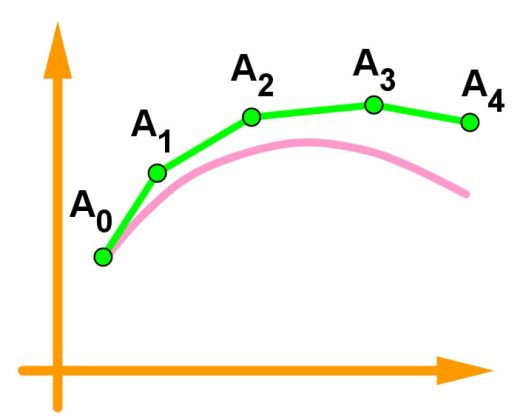Figure 1. Graphic representation of the Euler's method

As you can see from figure 1, Euler's method meaningful approach comes through a graphic representation of the problem we are solving. This graph will show us the closeness to a real value for a problem which we already know the answer, and it will give us confidence that in the case we have an "unsolvable" or so far unknown problem, we can get very close to its solution by using this approach.

For all practical purposes, as mentioned before, Euler's method is rarely used to solve differential equations by hand since we truly have other mathematical techniques which can help us out in accurately solving these kind of problems. Although later on this article we will talk about our advantages nowadays in using Euler's method through computational science, remember that this is usually a technique left for one of those cases where you cannot find the perfect solution, or the solution is completely unknown and after trying with other methods you are not obtaining meaningful answers.

In order for us to learn Euler's method at this time, we will have to work it out through already known problems so you can see the validity of the approach and its usefulness in case of utilizing it as a last resort in a real life problem. For this, let us solve the next initial value problem first by obtaining the answer with the method of separable equations in which you separate the variables and integrate them individually, and then we will confirm the result using Euler's technique. Therefore, for the following initial value problem:

• We first solve it using the method of separable equations: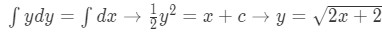Question 1: solve problem using separable equations pt.1
• Then in order to obtain the value of "c" we substitute the final equation with the initial values, in this case, when x=0, then y=1. So the equation looks and solves as follows: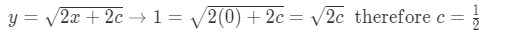Question 1: solve problem using separable equations pt.2
So the solution for this initial value problem is: $y=\sqrt{2x+1}$
• Let us plot this in the next graph for the values in the next table: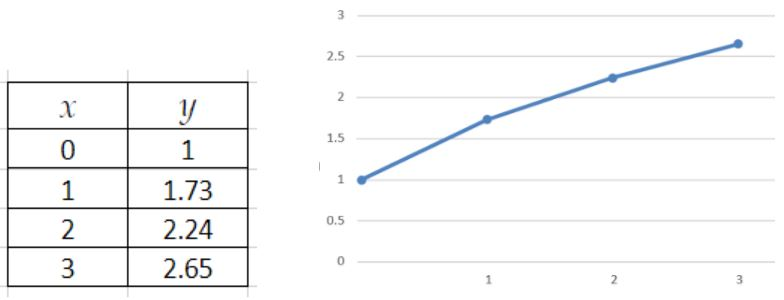Figure 2. Table and graph for values of y found through separable equations method
• Now let us work through the same problem but this time as an Euler's method example, and see how the result will be approximated to its real solution. Thus, going back to the initial conditions: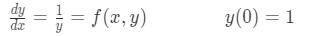Question 1: solve problem using separable equations pt.4
• Using a step size of h=0.5, we will find the approximate solution to y(1), y(2) and y(3) using the linear approximation formula: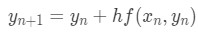Question 1: solve problem using separable equations pt.5
• As a result,we will fill the next table with the appropriate values: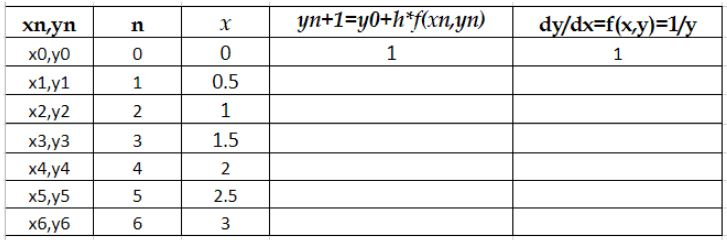Figure 3. Table to fill with y and dy/dx values using Euler's method
• Now let us solve the Euler's formula for all the values of n wanted: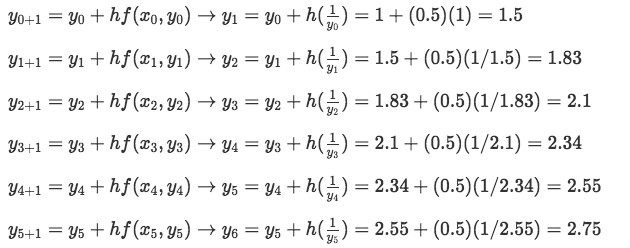Question 1: solve problem using separable equations pt.7
• Having the values for $y_n$ now we just compute the values of $\frac{dy}{dx}=f(x_n,y_n)=\frac{1}{y}$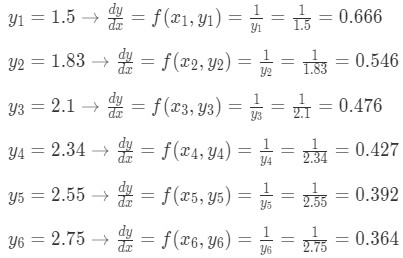Question 1: solve problem using separable equations pt.8
• And so, we fill the graph with the obtained values: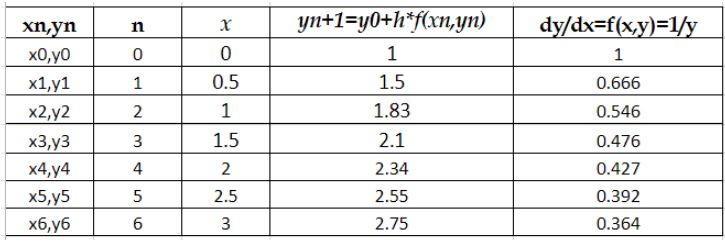Figure 4. Filled table with y and dy/dx values using Euler's method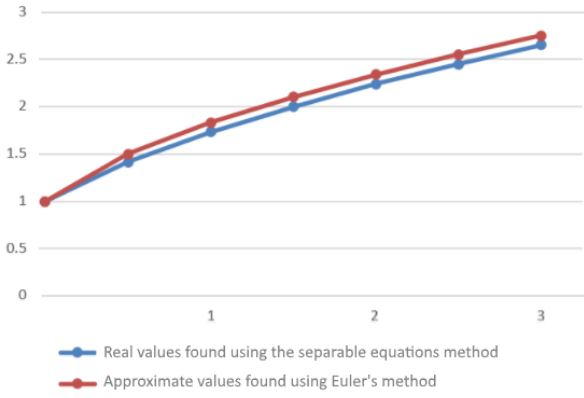Figure 5. Result comparison from the two methods mentioned before

As you can see from the tables and comparison graph, although Euler's method gives us a pretty good idea of the solution to a differential equation, it gets heavy on the computation side, and since is not completely accurate, we leave this technique as a tool at hand to problems we cannot obtain an answer with other methods analytical methods.

An important detail to notice is in the last column of the table in figure 4. The values of the derivative, notice how they decrease as we continue further on x. These make sense since the derivative represents the slope of the tangent line at each point in the graph, which is visibly decreasing as the function expands on the positive x direction.

To add to your practice and knowledge you can check out the video on top talking about how to solve the first few points in the table for the example we just went through. Make sure you follow the problem step by step, throughout this article you can go back and check all of your calculations in case you have any doubt.

## So what is Euler's method precisely?

In simple terms the Euler's method is a clever mathematical trick to solve "impossible" differential equations. The word impossible here is being used to denote those differential equations which people have tried and haven't found a nice solution using analytical methods, so the best thing to do is to find the closest answer to their real solution. Something nice to note is that although Leonhard Euler came up with his method in the late 1700's, its importance has actually got more noticeable with time due the amount of computations needed to obtain more accurate values for the function in question.

What does that mean? Well, as you can see from the little example on top, the values we obtain through the Euler's method, although they are close enough to give us an idea of the behavior of the function there is still an error margin associated to our solution. In order to obtain a higher amount of information for the function we would need to add as many more step points for the independent variable x on the table, and to decrease the size of the step.

The reason behind this is that we are changing the direction of the tangent line at every step we define in our approximation, in order to keep up with the continuously changing line of the real function, we will want to change the direction as often as possible to try to "catch up" with the real change. As we define our step size smaller, we are creating more steps to compute for the same size of the function in question. This would result in an increasingly large amount of computations that would have to be made by hand in order to produce a much accurate answer.

Thus, in order to get approximations as close as possible to real values one would have to work through hundreds, if not more, calculations which are more efficiently arrived to with the use of computers. For this reason, Euler's method is a great tool in the modern times which is usually worked through computer codes to obtain the solutions for hundreds, if not thousands or more, values for a function with step sizes that are small enough for us to get much meaningful solutions than the ones we can compute by hand.

Let us reiterate this, Euler's method is easily done by hand, the complication comes from the amount of values and thus computations one has to make to get as close as possible to the real values, therefore, on this day and era we take approach of a computer's speed for making the calculations for us instead, and in this manner, to solve problems faster which would take us much longer if we were to compute them by hand. After all, that is where the name "computer" came from, thanks to the rapidness and efficiency of the machine at "computing".

If you are curious as to how this method is used with code-processing mathematical software such as matlab, we recommend you to take a look at the example found in these differential equations notes where you will be able to not only go ahead and re-check Euler's methodology step by step, but also to take a look at a real-life example of a computer code to solve a differential equation problem through this technique.

## Solve the initial value problem:

Now let us work through another example. We first need to go back to the formulae of Euler's method differential equations: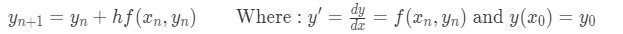formulae of Euler's method differential equations
And having the next initial values: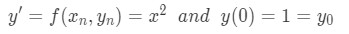Question 2: find the initial values using Euler's method
• Using a step size $h=0.2$ we compute the approximate values of $y$ to $y(1)$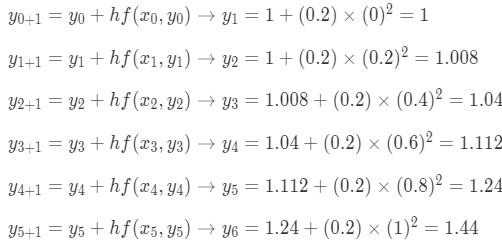Question 2: find the initial values using Euler's method pt.1
• We also compute the values of the derivative by themselves: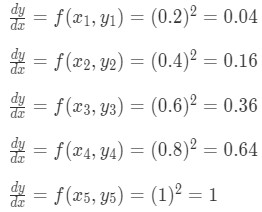Question 2: find the initial values using Euler's method pt.2
• So we can complete the next table already: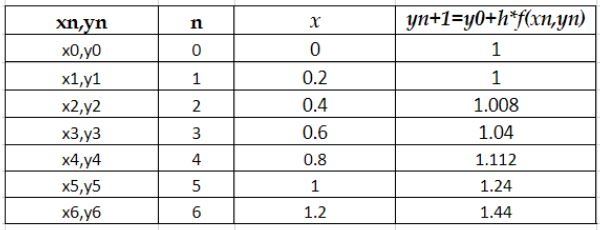Figure 6. Example of values found through Euler's method
• Having these values, we solve the exact same problem using the separable equations method to see the approximation we achieved: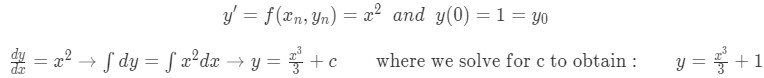Question 2: find the initial values using Euler's method pt.4
• And so we obtain the values of x and y using the found equation and fill up the next table: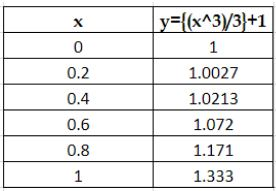Figure 7. Result values using method of separable equations
• Now let us plot both results together to see the comparison between the values obtained: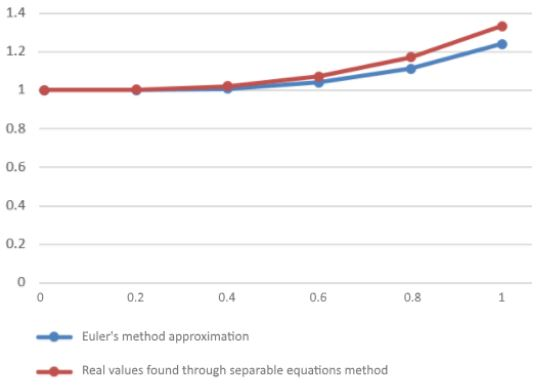Figure 8. Comparison of results from Euler's and Separable Equations method up to y(x=1)

### Euler's method

#### Lessons

So far we've learned how to solve many different differential equations. But actually there is a wide assortment of differential equations that will not be explicitly solvable with any of the techniques we've learned (or could learn).

So for this section we will focus on figuring out an approximation to differential equations that we are not able to explicitly solve.

A useful tool we will be able to use is Slope Fields.

There is a wide variety of numerical methods that approximate the solution to a differential equation, for now we will focus on Euler's Method.

Euler's Method:

To use Euler's Method we require the following:

$\cdot$ A Differential Equation (we can't solve)
$\frac{dy}{dx}=f(x,y)$
$\cdot$ Initial Conditions: $y(x_0 )=y_0$
$\cdot$ A step size $h$~step size

$y_{n+1}=y_n+h*f(x_n,y_n)$

$Error=\frac{|exact\;-\;approx.|}{exact} \times 100$%
• Introduction
a)
Error using Euler's Method

• 1.
Determining how to use Euler's Method
For the following Initial Value Problem

$\frac{dy}{dx}=\frac{1}{y}$
$y(0)=1$

Using Euler's Method with a step size of $h$=0.5 find the approximate solution to $y(1)$

• 2.
Estimating the Solution to a Differential Equation Using Euler's Method
For the following Initial Value Problem

$\frac{dy}{dx}=y^3-\frac{1}{x}y$
$y(\frac{1}{2})=1$
a)
Using Euler's Method with a step size of $h=1$ find the approximate solution to the value of $y$ at $x=1.5$

b)
Using Euler's Method with a step size of $h=0.25$ find the approximate solution to the value of $y$ at $x=1.5$

c)
The explicit solution to the above equation satisfying the initial conditions is $y=\frac{1}{\sqrt{2x}}$. Compare the error found in the previous two parts.